### 4.3.4.3. three parameters/one final graph

Proposition 68

$\begin{array}{cc}& \mathrm{𝐌𝐈𝐍}_\mathrm{𝐍𝐂𝐂}\ne \mathrm{𝐌𝐀𝐗}_\mathrm{𝐍𝐂𝐂}⇒\hfill \\ & \mathrm{𝐍𝐀𝐑𝐂}\ge \mathrm{𝐌𝐈𝐍}_\mathrm{𝐍𝐂𝐂}+\mathrm{𝐌𝐀𝐗}_\mathrm{𝐍𝐂𝐂}-2+\left(\mathrm{𝐌𝐈𝐍}_\mathrm{𝐍𝐂𝐂}=1\right)\hfill \end{array}$

$\begin{array}{cc}& \mathrm{𝚎𝚚𝚞𝚒𝚟𝚊𝚕𝚎𝚗𝚌𝚎}:\mathrm{𝐌𝐈𝐍}_\mathrm{𝐍𝐂𝐂}\ne \mathrm{𝐌𝐀𝐗}_\mathrm{𝐍𝐂𝐂}⇒\hfill \\ & \mathrm{𝐍𝐀𝐑𝐂}\ge \mathrm{𝐌𝐈𝐍}_{\mathrm{𝐍𝐂𝐂}}^{2}+\mathrm{𝐌𝐀𝐗}_{\mathrm{𝐍𝐂𝐂}}^{2}\hfill \end{array}$

Proof 68 (89) $n-1$ arcs are needed to connect $n$ $\left(n>1\right)$ vertices that all belong to a given connected component. Since we have two connected components, which respectively have $\mathrm{𝐌𝐈𝐍}_\mathrm{𝐍𝐂𝐂}$ and $\mathrm{𝐌𝐀𝐗}_\mathrm{𝐍𝐂𝐂}$ vertices, this leads to the previous inequality. When $\mathrm{𝐌𝐈𝐍}_\mathrm{𝐍𝐂𝐂}$ is equal to one we need an extra arc.

Proposition 69

$\mathrm{𝐌𝐈𝐍}_\mathrm{𝐍𝐂𝐂}\ne \mathrm{𝐌𝐀𝐗}_\mathrm{𝐍𝐂𝐂}⇒\mathrm{𝐍𝐂𝐂}\ge 2$

Proposition 70

$\mathrm{𝐌𝐈𝐍}_\mathrm{𝐍𝐂𝐂}\ne \mathrm{𝐌𝐀𝐗}_\mathrm{𝐍𝐂𝐂}⇒\mathrm{𝐍𝐕𝐄𝐑𝐓𝐄𝐗}\ge \mathrm{𝐌𝐈𝐍}_\mathrm{𝐍𝐂𝐂}+\mathrm{𝐌𝐀𝐗}_\mathrm{𝐍𝐂𝐂}$

Proof 70 Since we have at least two distinct connected components, which respectively have $\mathrm{𝐌𝐈𝐍}_\mathrm{𝐍𝐂𝐂}$ and $\mathrm{𝐌𝐀𝐗}_\mathrm{𝐍𝐂𝐂}$ vertices, this leads to the previous inequality.

Proposition 71

$\mathrm{𝐌𝐀𝐗}_\mathrm{𝐍𝐂𝐂}\le max\left(\mathrm{𝐌𝐈𝐍}_\mathrm{𝐍𝐂𝐂},\mathrm{𝐍𝐕𝐄𝐑𝐓𝐄𝐗}-max\left(1,\mathrm{𝐌𝐈𝐍}_\mathrm{𝐍𝐂𝐂}\right)\right)$

Proof 71 On the one hand, if $\mathrm{𝐍𝐂𝐂}\le 1$, we have that $\mathrm{𝐌𝐀𝐗}_\mathrm{𝐍𝐂𝐂}\le \mathrm{𝐌𝐈𝐍}_\mathrm{𝐍𝐂𝐂}$. On the other hand, if $\mathrm{𝐍𝐂𝐂}>1$, we have that $\mathrm{𝐍𝐕𝐄𝐑𝐓𝐄𝐗}\ge max\left(1,\mathrm{𝐌𝐈𝐍}_\mathrm{𝐍𝐂𝐂}\right)+\mathrm{𝐌𝐀𝐗}_\mathrm{𝐍𝐂𝐂}$ (i.e., $\mathrm{𝐌𝐀𝐗}_\mathrm{𝐍𝐂𝐂}\le \mathrm{𝐍𝐕𝐄𝐑𝐓𝐄𝐗}-max\left(1,\mathrm{𝐌𝐈𝐍}_\mathrm{𝐍𝐂𝐂}\right)$). The result is obtained by taking the maximum value of the right-hand sides of the two inequalities.

Proposition 72

$\mathrm{𝐌𝐈𝐍}_\mathrm{𝐍𝐂𝐂}\notin \left[\mathrm{𝐍𝐕𝐄𝐑𝐓𝐄𝐗}-max\left(1,\mathrm{𝐌𝐀𝐗}_\mathrm{𝐍𝐂𝐂}\right)+1,\mathrm{𝐍𝐕𝐄𝐑𝐓𝐄𝐗}-1\right]$

Proof 72 On the one hand, if $\mathrm{𝐍𝐂𝐂}\le 1$, we have that $\mathrm{𝐌𝐈𝐍}_\mathrm{𝐍𝐂𝐂}\ge \mathrm{𝐍𝐕𝐄𝐑𝐓𝐄𝐗}$. On the other hand, if $\mathrm{𝐍𝐂𝐂}>1$, we have that $\mathrm{𝐌𝐈𝐍}_\mathrm{𝐍𝐂𝐂}+max\left(1,\mathrm{𝐌𝐀𝐗}_\mathrm{𝐍𝐂𝐂}\right)\le \mathrm{𝐍𝐕𝐄𝐑𝐓𝐄𝐗}$ (i.e., $\mathrm{𝐌𝐈𝐍}_\mathrm{𝐍𝐂𝐂}\le \mathrm{𝐍𝐕𝐄𝐑𝐓𝐄𝐗}-max\left(1,\mathrm{𝐌𝐀𝐗}_\mathrm{𝐍𝐂𝐂}\right)$). The result follows.

Proposition 73

$\mathrm{𝐍𝐕𝐄𝐑𝐓𝐄𝐗}\notin \left[\mathrm{𝐌𝐈𝐍}_\mathrm{𝐍𝐂𝐂}+1,\mathrm{𝐌𝐈𝐍}_\mathrm{𝐍𝐂𝐂}+\mathrm{𝐌𝐀𝐗}_\mathrm{𝐍𝐂𝐂}-1\right]$

Proof 73 On the one hand, if $\mathrm{𝐍𝐂𝐂}\le 1$, we have that $\mathrm{𝐍𝐕𝐄𝐑𝐓𝐄𝐗}\le \mathrm{𝐌𝐈𝐍}_\mathrm{𝐍𝐂𝐂}$. On the other hand, if $\mathrm{𝐍𝐂𝐂}>1$, we have that $\mathrm{𝐍𝐕𝐄𝐑𝐓𝐄𝐗}\ge \mathrm{𝐌𝐈𝐍}_\mathrm{𝐍𝐂𝐂}+\mathrm{𝐌𝐀𝐗}_\mathrm{𝐍𝐂𝐂}$. Since $\mathrm{𝐌𝐈𝐍}_\mathrm{𝐍𝐂𝐂}\le \mathrm{𝐌𝐈𝐍}_\mathrm{𝐍𝐂𝐂}+\mathrm{𝐌𝐀𝐗}_\mathrm{𝐍𝐂𝐂}$ the result follows.

Proposition 74

$\begin{array}{cc}& \mathrm{𝚒𝚏}\overline{\mathrm{𝐌𝐈𝐍}_\mathrm{𝐍𝐂𝐂}}>0\hfill \\ & \mathrm{𝚝𝚑𝚎𝚗}{k}_{\mathrm{𝑖𝑛𝑓}}=⌊\frac{\underline{\mathrm{𝐍𝐕𝐄𝐑𝐓𝐄𝐗}}+\overline{\mathrm{𝐌𝐈𝐍}_\mathrm{𝐍𝐂𝐂}}}{\overline{\mathrm{𝐌𝐈𝐍}_\mathrm{𝐍𝐂𝐂}}}⌋\mathrm{𝚎𝚕𝚜𝚎}{k}_{\mathrm{𝑖𝑛𝑓}}=1\hfill \end{array}$
$\begin{array}{cc}& \mathrm{𝚒𝚏}\underline{\mathrm{𝐌𝐀𝐗}_\mathrm{𝐍𝐂𝐂}}>0\hfill \\ & \mathrm{𝚝𝚑𝚎𝚗}{k}_{{\mathrm{𝑠𝑢𝑝}}_{1}}=⌊\frac{\overline{\mathrm{𝐍𝐕𝐄𝐑𝐓𝐄𝐗}}-1}{\underline{\mathrm{𝐌𝐀𝐗}_\mathrm{𝐍𝐂𝐂}}}⌋\mathrm{𝚎𝚕𝚜𝚎}{k}_{{\mathrm{𝑠𝑢𝑝}}_{1}}=\overline{\mathrm{𝐍𝐕𝐄𝐑𝐓𝐄𝐗}}\hfill \end{array}$
$\begin{array}{cc}& \mathrm{𝚒𝚏}\underline{\mathrm{𝐌𝐀𝐗}_\mathrm{𝐍𝐂𝐂}}<\overline{\mathrm{𝐌𝐈𝐍}_\mathrm{𝐍𝐂𝐂}}\hfill \\ & \mathrm{𝚝𝚑𝚎𝚗}{k}_{{\mathrm{𝑠𝑢𝑝}}_{2}}=⌊\frac{\overline{\mathrm{𝐌𝐈𝐍}_\mathrm{𝐍𝐂𝐂}}-2}{\underline{\mathrm{𝐌𝐀𝐗}_\mathrm{𝐍𝐂𝐂}}-\overline{\mathrm{𝐌𝐈𝐍}_\mathrm{𝐍𝐂𝐂}}}⌋\mathrm{𝚎𝚕𝚜𝚎}{k}_{{\mathrm{𝑠𝑢𝑝}}_{2}}=\overline{\mathrm{𝐍𝐕𝐄𝐑𝐓𝐄𝐗}}\hfill \end{array}$
${k}_{\mathrm{𝑠𝑢𝑝}}=min\left({k}_{{\mathrm{𝑠𝑢𝑝}}_{1}},{k}_{{\mathrm{𝑠𝑢𝑝}}_{2}}\right)$
$\forall k\in \left[{k}_{\mathrm{𝑖𝑛𝑓}},{k}_{\mathrm{𝑠𝑢𝑝}}\right]:\mathrm{𝐍𝐕𝐄𝐑𝐓𝐄𝐗}\notin \left[k·\mathrm{𝐌𝐀𝐗}_\mathrm{𝐍𝐂𝐂}+1,\left(k+1\right)·\mathrm{𝐌𝐈𝐍}_\mathrm{𝐍𝐂𝐂}-1\right]$

Proof 74 We make the proof for $k\in ℕ$ (the interval $\left[{k}_{\mathrm{𝑖𝑛𝑓}},{k}_{\mathrm{𝑠𝑢𝑝}}\right]$ is only used for restricting the number of intervals to check). We have that $\mathrm{𝐍𝐕𝐄𝐑𝐓𝐄𝐗}\in \left[k·\mathrm{𝐌𝐈𝐍}_\mathrm{𝐍𝐂𝐂},k·\mathrm{𝐌𝐀𝐗}_\mathrm{𝐍𝐂𝐂}\right]$. A forbidden interval $\left[k·\mathrm{𝐌𝐀𝐗}_\mathrm{𝐍𝐂𝐂}+1,\left(k+1\right)·\mathrm{𝐌𝐈𝐍}_\mathrm{𝐍𝐂𝐂}-1\right]$ corresponds to an interval between the end of interval $\left[k·\mathrm{𝐌𝐈𝐍}_\mathrm{𝐍𝐂𝐂},k·\mathrm{𝐌𝐀𝐗}_\mathrm{𝐍𝐂𝐂}\right]$ and the start of the next interval $\left[\left(k+1\right)·\mathrm{𝐌𝐈𝐍}_\mathrm{𝐍𝐂𝐂},\left(k+1\right)·\mathrm{𝐌𝐀𝐗}_\mathrm{𝐍𝐂𝐂}\right]$. Since all intervals $\left[i·\mathrm{𝐌𝐈𝐍}_\mathrm{𝐍𝐂𝐂},i·\mathrm{𝐌𝐀𝐗}_\mathrm{𝐍𝐂𝐂}\right]$ $\left(i end before $k·\mathrm{𝐌𝐀𝐗}_\mathrm{𝐍𝐂𝐂}$ and since all intervals $\left[j·\mathrm{𝐌𝐈𝐍}_\mathrm{𝐍𝐂𝐂},j·\mathrm{𝐌𝐀𝐗}_\mathrm{𝐍𝐂𝐂}\right]$ $\left(j>k\right)$ start after $\left(k+1\right)·\mathrm{𝐌𝐈𝐍}_\mathrm{𝐍𝐂𝐂}$, they do not use any value in $\left[k·\mathrm{𝐌𝐀𝐗}_\mathrm{𝐍𝐂𝐂}+1,\left(k+1\right)·\mathrm{𝐌𝐈𝐍}_\mathrm{𝐍𝐂𝐂}-1\right]$.

Proposition 75

$\mathrm{𝐍𝐀𝐑𝐂}\le \mathrm{𝐍𝐂𝐂}·\mathrm{𝐌𝐀𝐗}_{\mathrm{𝐍𝐂𝐂}}^{2}$

$\mathrm{𝐚𝐫𝐜}_\mathrm{𝐠𝐞𝐧}=\mathrm{𝑃𝐴𝑇𝐻}:\mathrm{𝐍𝐀𝐑𝐂}\le \mathrm{𝐍𝐂𝐂}·\left(\mathrm{𝐌𝐀𝐗}_\mathrm{𝐍𝐂𝐂}-1\right)$

Proof 75 On the one hand, (97) holds since the maximum number of arcs is achieved by taking $\mathrm{𝐍𝐂𝐂}$ connected components where each connected component is a clique involving $\mathrm{𝐌𝐀𝐗}_\mathrm{𝐍𝐂𝐂}$ vertices. On the other hand, (98) holds since a tree of $n$ vertices has $n-1$ arcs.

Proposition 76

$\mathrm{𝐍𝐀𝐑𝐂}\ge \mathrm{𝐌𝐀𝐗}_\mathrm{𝐍𝐂𝐂}+\mathrm{𝐍𝐂𝐂}-2$

Proof 76 The minimum number of arcs is achieved by taking one connected component with $\mathrm{𝐌𝐀𝐗}_\mathrm{𝐍𝐂𝐂}$ vertices and $\mathrm{𝐌𝐀𝐗}_\mathrm{𝐍𝐂𝐂}-1$ arcs as well as $\mathrm{𝐍𝐂𝐂}-1$ connected components with a single vertex and a loop.

Proposition 77

$\mathrm{𝐍𝐀𝐑𝐂}\le \mathrm{𝐌𝐀𝐗}_{\mathrm{𝐍𝐂𝐂}}^{2}·⌊\frac{\mathrm{𝐍𝐕𝐄𝐑𝐓𝐄𝐗}}{max\left(1,\mathrm{𝐌𝐀𝐗}_\mathrm{𝐍𝐂𝐂}\right)}⌋+{\left(\mathrm{𝐍𝐕𝐄𝐑𝐓𝐄𝐗}\mathrm{mod}max\left(1,\mathrm{𝐌𝐀𝐗}_\mathrm{𝐍𝐂𝐂}\right)\right)}^{2}$

##### Figure 4.3.1. Illustration of Proposition 77. A graph that achieves the maximum number of arcs according to the size of the largest connected component as well as to a fixed number of vertices ($\mathrm{𝙼𝙰𝚇}_\mathrm{𝙽𝙲𝙲}=3,\mathrm{𝙽𝚅𝙴𝚁𝚃𝙴𝚇}=11,\mathrm{𝙽𝙰𝚁𝙲}={3}^{2}·⌊\frac{11}{max\left(1,3\right)}⌋+{\left(11\mathrm{mod}max\left(1,3\right)\right)}^{2}=31$)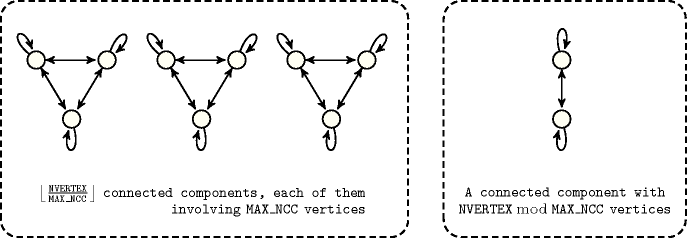Proof 77 If $\mathrm{𝐌𝐀𝐗}_\mathrm{𝐍𝐂𝐂}=0$ we get $\mathrm{𝐍𝐀𝐑𝐂}\le 0$ which holds since the set of vertices is empty. We now assume that $\mathrm{𝐌𝐀𝐗}_\mathrm{𝐍𝐂𝐂}>0$. We first begin with the following claim:

let $G$ be a graph such that $V\left(G\right)-\mathrm{𝐍𝐂𝐂}\left(G,\mathrm{𝐌𝐀𝐗}_\mathrm{𝐍𝐂𝐂}\left(G\right)\right)*\mathrm{𝐌𝐀𝐗}_\mathrm{𝐍𝐂𝐂}\left(G\right)\ge \mathrm{𝐌𝐀𝐗}_\mathrm{𝐍𝐂𝐂}\left(G\right)$, then there exists a graph ${G}^{\text{'}}$ such that $V\left({G}^{\text{'}}\right)=V\left(G\right)$, $\mathrm{𝐌𝐀𝐗}_\mathrm{𝐍𝐂𝐂}\left({G}^{\text{'}}\right)=\mathrm{𝐌𝐀𝐗}_\mathrm{𝐍𝐂𝐂}\left(G\right)$, $\mathrm{𝐍𝐂𝐂}\left({G}^{\text{'}},\mathrm{𝐌𝐀𝐗}_\mathrm{𝐍𝐂𝐂}\left({G}^{\text{'}}\right)\right)=\mathrm{𝐍𝐂𝐂}\left(G,\mathrm{𝐌𝐀𝐗}_\mathrm{𝐍𝐂𝐂}\left(G\right)\right)+1$ and $|E\left(G\right)|\le |E\left({G}^{\text{'}}\right)|$.

Proof of the claim

Let ${\left({C}_{i}\right)}_{i\in \left[n\right]}$ be the connected components of $G$ on less than $\mathrm{𝐌𝐀𝐗}_\mathrm{𝐍𝐂𝐂}\left(G\right)$ vertices and such that $|{C}_{i}|\ge |{C}_{i+1}|$. By hypothesis there exists $k\le n$ such that $|{\bigcup }_{i=1}^{k-1}{C}_{i}|<\mathrm{𝐌𝐀𝐗}_\mathrm{𝐍𝐂𝐂}\left(G\right)$ and $|{\bigcup }_{i=1}^{k}{C}_{i}|\ge \mathrm{𝐌𝐀𝐗}_\mathrm{𝐍𝐂𝐂}\left(G\right)$.

• Either $|{\bigcup }_{i=1}^{k}{C}_{i}|=\mathrm{𝐌𝐀𝐗}_\mathrm{𝐍𝐂𝐂}\left(G\right)$, and then with ${G}^{\text{'}}$ such that ${G}^{\text{'}}$ restricted to the ${\bigcup }_{i=1}^{k}{C}_{i}$ be a complete graph and ${G}^{\text{'}}$ restricted to $V\left(G\right)-{\bigcup }_{i=1}^{k}{C}_{i}$ being exactly $G$ restricted to $V\left(G\right)-{\bigcup }_{i=1}^{k}{C}_{i}$ we obtain the claim.

• Or $|{\bigcup }_{i=1}^{k}{C}_{i}|>\mathrm{𝐌𝐀𝐗}_\mathrm{𝐍𝐂𝐂}\left(G\right)$. Then ${C}_{k}={C}_{k}^{1}\uplus {C}_{k}^{2}$ such that $|\left({\bigcup }_{i=1}^{k-1}{C}_{i}\right)\cup {C}_{k}^{1}|=\mathrm{𝐌𝐀𝐗}_\mathrm{𝐍𝐂𝐂}\left(G\right)$ and $|{C}_{k}^{2}|<|{C}_{1}|$ (notice that $k\ge 2$). Then with ${G}^{\text{'}}$ such that ${G}^{\text{'}}$ restricted to $\left({\bigcup }_{i=1}^{k-1}{C}_{i}\right)\cup {C}_{k}^{1}$ is a complete graph and ${G}^{\text{'}}$ restricted to $V\left(G\right)-\left(\left({\bigcup }_{i=1}^{k-1}{C}_{i}\right)\cup {C}_{k}^{1}\right)$ is exactly $G$ restricted to $V\left(G\right)-\left(\left({\bigcup }_{i=1}^{k-1}{C}_{i}\right)\cup {C}_{k}^{1}\right)$ we obtain the claim.

End of proof of the claim

We prove by induction on $r\left(G\right)=⌊\frac{\mathrm{𝐍𝐕𝐄𝐑𝐓𝐄𝐗}\left(G\right)}{\mathrm{𝐌𝐀𝐗}_\mathrm{𝐍𝐂𝐂}\left(G\right)}⌋-\mathrm{𝐍𝐂𝐂}\left(G,\mathrm{𝐌𝐀𝐗}_\mathrm{𝐍𝐂𝐂}\left(G\right)\right)$, where $G$ is any graph. For $r\left(G\right)=0$ the result holds (see Prop 44). Otherwise, since $r\left(G\right)>0$ we have that $V\left(G\right)-\mathrm{𝐍𝐂𝐂}\left(G,\mathrm{𝐌𝐀𝐗}_\mathrm{𝐍𝐂𝐂}\left(G\right)\right)*\mathrm{𝐌𝐀𝐗}_\mathrm{𝐍𝐂𝐂}\left(G\right)\ge \mathrm{𝐌𝐀𝐗}_\mathrm{𝐍𝐂𝐂}\left(G\right)$, by the previous claim there exists ${G}^{\text{'}}$ with the same number of vertices and the same number of vertices in the largest connected component, such that $r\left({G}^{\text{'}}\right)=r\left(G\right)-1$. Consequently the result holds by induction.

Proposition 78

$\mathrm{𝐍𝐀𝐑𝐂}\ge \mathrm{𝐌𝐀𝐗}_\mathrm{𝐍𝐂𝐂}-1+⌊\frac{\mathrm{𝐍𝐕𝐄𝐑𝐓𝐄𝐗}-\mathrm{𝐌𝐀𝐗}_\mathrm{𝐍𝐂𝐂}+1}{2}⌋$

Proof 78 Let $G$ be a graph, let $X$ be a maximal size connected component of $G$, then we have $G=G\left[X\right]\oplus G\left[V\left(G\right)-X\right]$. On the one hand, as $G\left[X\right]$ is connected, by setting $\mathrm{𝐍𝐂𝐂}=1$ in 143 of Proposition 99, we have $|E\left(G\left[X\right]\right)\ge |X|-1$, on the other hand, by Proposition 52, $|E\left(G\left[V\left(G\right)-X\right]\right)|\ge ⌈\frac{|V\left(G\right)-X|}{2}⌉$. Thus the result follows.

Proposition 79

$\mathrm{𝐍𝐒𝐈𝐍𝐊}\le \mathrm{𝐍𝐂𝐂}·max\left(0,\mathrm{𝐌𝐀𝐗}_\mathrm{𝐍𝐂𝐂}-1\right)$

Proof 79 Since a connected component contains at most $\mathrm{𝐌𝐀𝐗}_\mathrm{𝐍𝐂𝐂}$ vertices and since it does not contain any isolated vertex a connected component involves at most $\mathrm{𝐌𝐀𝐗}_\mathrm{𝐍𝐂𝐂}-1$ sinks. Thus the result follows.

Proposition 80

$\mathrm{𝐍𝐒𝐎𝐔𝐑𝐂𝐄}\le \mathrm{𝐍𝐂𝐂}·max\left(0,\mathrm{𝐌𝐀𝐗}_\mathrm{𝐍𝐂𝐂}-1\right)$

Proof 80 Similar to Proposition 79.

Proposition 81

$\mathrm{𝐍𝐕𝐄𝐑𝐓𝐄𝐗}\le \mathrm{𝐍𝐂𝐂}·\mathrm{𝐌𝐀𝐗}_\mathrm{𝐍𝐂𝐂}$

Proof 81 The number of vertices is less than or equal to the number of connected components multiplied by the largest number of vertices in a connected component.

Proposition 82

$\mathrm{𝐍𝐕𝐄𝐑𝐓𝐄𝐗}\ge \mathrm{𝐌𝐀𝐗}_\mathrm{𝐍𝐂𝐂}+max\left(0,\mathrm{𝐍𝐂𝐂}-1\right)$

$\mathrm{𝚗𝚘}\mathrm{𝚕𝚘𝚘𝚙}:\mathrm{𝐍𝐕𝐄𝐑𝐓𝐄𝐗}\ge \mathrm{𝐌𝐀𝐗}_\mathrm{𝐍𝐂𝐂}+max\left(0,2·\mathrm{𝐍𝐂𝐂}-2\right)$

Proof 82 (105) The minimum number of vertices according to a fixed number of connected components $\mathrm{𝐍𝐂𝐂}$ such that one of the connected components contains $\mathrm{𝐌𝐀𝐗}_\mathrm{𝐍𝐂𝐂}$ vertices is obtained as follows: we get $\mathrm{𝐌𝐀𝐗}_\mathrm{𝐍𝐂𝐂}$ vertices from the connected component involving $\mathrm{𝐌𝐀𝐗}_\mathrm{𝐍𝐂𝐂}$ vertices and one vertex for each remaining connected component.

Proposition 83

$\mathrm{𝐌𝐈𝐍}_\mathrm{𝐍𝐒𝐂𝐂}\ne \mathrm{𝐌𝐀𝐗}_\mathrm{𝐍𝐒𝐂𝐂}⇒\mathrm{𝐍𝐀𝐑𝐂}\ge \mathrm{𝐌𝐈𝐍}_\mathrm{𝐍𝐒𝐂𝐂}+\mathrm{𝐌𝐀𝐗}_\mathrm{𝐍𝐒𝐂𝐂}$

$\begin{array}{cc}& \mathrm{𝚎𝚚𝚞𝚒𝚟𝚊𝚕𝚎𝚗𝚌𝚎}:\mathrm{𝐌𝐈𝐍}_\mathrm{𝐍𝐒𝐂𝐂}\ne \mathrm{𝐌𝐀𝐗}_\mathrm{𝐍𝐒𝐂𝐂}⇒\hfill \\ & \mathrm{𝐍𝐀𝐑𝐂}\ge \mathrm{𝐌𝐈𝐍}_{\mathrm{𝐍𝐒𝐂𝐂}}^{2}+\mathrm{𝐌𝐀𝐗}_{\mathrm{𝐍𝐒𝐂𝐂}}^{2}\hfill \end{array}$

Proof 83 (107) In a strongly connected component at least one arc has to leave each arc. Since we have two strongly connected components, which respectively have $\mathrm{𝐌𝐈𝐍}_\mathrm{𝐍𝐒𝐂𝐂}$ and $\mathrm{𝐌𝐀𝐗}_\mathrm{𝐍𝐒𝐂𝐂}$ vertices, this leads to the previous inequality.

Proposition 84

$\mathrm{𝐌𝐈𝐍}_\mathrm{𝐍𝐒𝐂𝐂}\ne \mathrm{𝐌𝐀𝐗}_\mathrm{𝐍𝐒𝐂𝐂}⇒\mathrm{𝐍𝐒𝐂𝐂}\ge 2$

Proposition 85

$\mathrm{𝐌𝐈𝐍}_\mathrm{𝐍𝐒𝐂𝐂}\ne \mathrm{𝐌𝐀𝐗}_\mathrm{𝐍𝐒𝐂𝐂}⇒\mathrm{𝐍𝐕𝐄𝐑𝐓𝐄𝐗}\ge \mathrm{𝐌𝐈𝐍}_\mathrm{𝐍𝐒𝐂𝐂}+\mathrm{𝐌𝐀𝐗}_\mathrm{𝐍𝐒𝐂𝐂}$

Proof 85 Since we have at least two distinct strongly connected components, which respectively have $\mathrm{𝐌𝐈𝐍}_\mathrm{𝐍𝐒𝐂𝐂}$ and $\mathrm{𝐌𝐀𝐗}_\mathrm{𝐍𝐒𝐂𝐂}$ vertices, this leads to the previous inequality.

Proposition 86

$\begin{array}{cc}& \mathrm{𝚒𝚏}\overline{\mathrm{𝐌𝐈𝐍}_\mathrm{𝐍𝐒𝐂𝐂}}>0\hfill \\ & \mathrm{𝚝𝚑𝚎𝚗}{k}_{\mathrm{𝑖𝑛𝑓}}=⌊\frac{\underline{\mathrm{𝐍𝐕𝐄𝐑𝐓𝐄𝐗}}+\overline{\mathrm{𝐌𝐈𝐍}_\mathrm{𝐍𝐒𝐂𝐂}}}{\overline{\mathrm{𝐌𝐈𝐍}_\mathrm{𝐍𝐒𝐂𝐂}}}⌋\mathrm{𝚎𝚕𝚜𝚎}{k}_{\mathrm{𝑖𝑛𝑓}}=1\hfill \end{array}$
$\begin{array}{cc}& \mathrm{𝚒𝚏}\underline{\mathrm{𝐌𝐀𝐗}_\mathrm{𝐍𝐒𝐂𝐂}}>0\hfill \\ & \mathrm{𝚝𝚑𝚎𝚗}{k}_{{\mathrm{𝑠𝑢𝑝}}_{1}}=⌊\frac{\overline{\mathrm{𝐍𝐕𝐄𝐑𝐓𝐄𝐗}}-1}{\underline{\mathrm{𝐌𝐀𝐗}_\mathrm{𝐍𝐒𝐂𝐂}}}⌋\mathrm{𝚎𝚕𝚜𝚎}{k}_{{\mathrm{𝑠𝑢𝑝}}_{1}}=\overline{\mathrm{𝐍𝐕𝐄𝐑𝐓𝐄𝐗}}\hfill \end{array}$
$\begin{array}{cc}& \mathrm{𝚒𝚏}\underline{\mathrm{𝐌𝐀𝐗}_\mathrm{𝐍𝐒𝐂𝐂}}<\overline{\mathrm{𝐌𝐈𝐍}_\mathrm{𝐍𝐒𝐂𝐂}}\hfill \\ & \mathrm{𝚝𝚑𝚎𝚗}{k}_{{\mathrm{𝑠𝑢𝑝}}_{2}}=⌊\frac{\overline{\mathrm{𝐌𝐈𝐍}_\mathrm{𝐍𝐒𝐂𝐂}}-2}{\underline{\mathrm{𝐌𝐀𝐗}_\mathrm{𝐍𝐒𝐂𝐂}}-\overline{\mathrm{𝐌𝐈𝐍}_\mathrm{𝐍𝐒𝐂𝐂}}}⌋\mathrm{𝚎𝚕𝚜𝚎}{k}_{{\mathrm{𝑠𝑢𝑝}}_{2}}=\overline{\mathrm{𝐍𝐕𝐄𝐑𝐓𝐄𝐗}}\hfill \end{array}$
${k}_{\mathrm{𝑠𝑢𝑝}}=min\left({k}_{{\mathrm{𝑠𝑢𝑝}}_{1}},{k}_{{\mathrm{𝑠𝑢𝑝}}_{2}}\right)$
$\forall k\in \left[{k}_{\mathrm{𝑖𝑛𝑓}},{k}_{\mathrm{𝑠𝑢𝑝}}\right]:\mathrm{𝐍𝐕𝐄𝐑𝐓𝐄𝐗}\notin \left[k·\mathrm{𝐌𝐀𝐗}_\mathrm{𝐍𝐒𝐂𝐂}+1,\left(k+1\right)·\mathrm{𝐌𝐈𝐍}_\mathrm{𝐍𝐒𝐂𝐂}-1\right]$

Proof 86 Similar to Proposition 74.

Proposition 87

$\mathrm{𝐍𝐕𝐄𝐑𝐓𝐄𝐗}\le \mathrm{𝐍𝐂𝐂}·\mathrm{𝐌𝐀𝐗}_\mathrm{𝐍𝐒𝐂𝐂}$

Proof 87 The largest number of vertices is obtained by putting within each connected component the number of vertices of the largest strongly connected component.

Proposition 88

$\mathrm{𝐍𝐕𝐄𝐑𝐓𝐄𝐗}\le \mathrm{𝐍𝐒𝐂𝐂}·\mathrm{𝐌𝐀𝐗}_\mathrm{𝐍𝐒𝐂𝐂}$

Proof 88 Since each strongly connected component contains at most $\mathrm{𝐌𝐀𝐗}_\mathrm{𝐍𝐒𝐂𝐂}$ vertices the total number of vertices is less than or equal to $\mathrm{𝐍𝐒𝐂𝐂}·\mathrm{𝐌𝐀𝐗}_\mathrm{𝐍𝐒𝐂𝐂}$.

Proposition 89

$\mathrm{𝐍𝐕𝐄𝐑𝐓𝐄𝐗}\ge \mathrm{𝐌𝐀𝐗}_\mathrm{𝐍𝐒𝐂𝐂}+max\left(0,\mathrm{𝐍𝐒𝐂𝐂}-1\right)$

$\mathrm{𝚗𝚘}\mathrm{𝚕𝚘𝚘𝚙}:\mathrm{𝐍𝐕𝐄𝐑𝐓𝐄𝐗}\ge \mathrm{𝐌𝐀𝐗}_\mathrm{𝐍𝐒𝐂𝐂}+max\left(0,2·\mathrm{𝐍𝐒𝐂𝐂}-2\right)$

Proof 89 (114) The minimum number of vertices according to a fixed number of strongly connected components $\mathrm{𝐍𝐒𝐂𝐂}$ such that one of them contains $\mathrm{𝐌𝐀𝐗}_\mathrm{𝐍𝐒𝐂𝐂}$ vertices is equal to $\mathrm{𝐌𝐀𝐗}_\mathrm{𝐍𝐒𝐂𝐂}+max\left(0,\mathrm{𝐍𝐒𝐂𝐂}-1\right)$.

Proposition 90

$\mathrm{𝐍𝐀𝐑𝐂}\le \mathrm{𝐌𝐈𝐍}_{\mathrm{𝐍𝐂𝐂}}^{2}+{\left(\mathrm{𝐍𝐕𝐄𝐑𝐓𝐄𝐗}-\mathrm{𝐌𝐈𝐍}_\mathrm{𝐍𝐂𝐂}\right)}^{2}$

$\mathrm{𝐚𝐫𝐜}_\mathrm{𝐠𝐞𝐧}=\mathrm{𝐶𝐼𝑅𝐶𝑈𝐼𝑇}:\mathrm{𝐍𝐀𝐑𝐂}\le \mathrm{𝐍𝐕𝐄𝐑𝐓𝐄𝐗}-2·\left(\mathrm{𝐌𝐈𝐍}_\mathrm{𝐍𝐂𝐂}<\mathrm{𝐍𝐕𝐄𝐑𝐓𝐄𝐗}\right)$

$\mathrm{𝐚𝐫𝐜}_\mathrm{𝐠𝐞𝐧}=\mathrm{𝐶𝐻𝐴𝐼𝑁}:\mathrm{𝐍𝐀𝐑𝐂}\le \mathrm{𝐍𝐕𝐄𝐑𝐓𝐄𝐗}-2·\left(\mathrm{𝐌𝐈𝐍}_\mathrm{𝐍𝐂𝐂}<\mathrm{𝐍𝐕𝐄𝐑𝐓𝐄𝐗}\right)$

$\begin{array}{cc}& \mathrm{𝐚𝐫𝐜}_\mathrm{𝐠𝐞𝐧}=\mathrm{𝐶𝐿𝐼𝑄𝑈𝐸}\left(\le \right):\mathrm{𝐍𝐀𝐑𝐂}\le \frac{\mathrm{𝐌𝐈𝐍}_\mathrm{𝐍𝐂𝐂}·\left(\mathrm{𝐌𝐈𝐍}_\mathrm{𝐍𝐂𝐂}+1\right)}{2}+\hfill \\ & \frac{\left(\mathrm{𝐍𝐕𝐄𝐑𝐓𝐄𝐗}-\mathrm{𝐌𝐈𝐍}_\mathrm{𝐍𝐂𝐂}\right)·\left(\mathrm{𝐍𝐕𝐄𝐑𝐓𝐄𝐗}-\mathrm{𝐌𝐈𝐍}_\mathrm{𝐍𝐂𝐂}+1\right)}{2}\hfill \end{array}$

$\begin{array}{cc}& \mathrm{𝐚𝐫𝐜}_\mathrm{𝐠𝐞𝐧}=\mathrm{𝐶𝐿𝐼𝑄𝑈𝐸}\left(\ge \right):\mathrm{𝐍𝐀𝐑𝐂}\le \frac{\mathrm{𝐌𝐈𝐍}_\mathrm{𝐍𝐂𝐂}·\left(\mathrm{𝐌𝐈𝐍}_\mathrm{𝐍𝐂𝐂}+1\right)}{2}+\hfill \\ & \frac{\left(\mathrm{𝐍𝐕𝐄𝐑𝐓𝐄𝐗}-\mathrm{𝐌𝐈𝐍}_\mathrm{𝐍𝐂𝐂}\right)·\left(\mathrm{𝐍𝐕𝐄𝐑𝐓𝐄𝐗}-\mathrm{𝐌𝐈𝐍}_\mathrm{𝐍𝐂𝐂}+1\right)}{2}\hfill \end{array}$

$\begin{array}{cc}& \mathrm{𝐚𝐫𝐜}_\mathrm{𝐠𝐞𝐧}=\mathrm{𝐶𝐿𝐼𝑄𝑈𝐸}\left(<\right):\mathrm{𝐍𝐀𝐑𝐂}\le \frac{\mathrm{𝐌𝐈𝐍}_\mathrm{𝐍𝐂𝐂}·\left(\mathrm{𝐌𝐈𝐍}_\mathrm{𝐍𝐂𝐂}-1\right)}{2}+\hfill \\ & \frac{\left(\mathrm{𝐍𝐕𝐄𝐑𝐓𝐄𝐗}-\mathrm{𝐌𝐈𝐍}_\mathrm{𝐍𝐂𝐂}\right)·\left(\mathrm{𝐍𝐕𝐄𝐑𝐓𝐄𝐗}-\mathrm{𝐌𝐈𝐍}_\mathrm{𝐍𝐂𝐂}-1\right)}{2}\hfill \end{array}$

$\begin{array}{cc}& \mathrm{𝐚𝐫𝐜}_\mathrm{𝐠𝐞𝐧}=\mathrm{𝐶𝐿𝐼𝑄𝑈𝐸}\left(>\right):\mathrm{𝐍𝐀𝐑𝐂}\le \frac{\mathrm{𝐌𝐈𝐍}_\mathrm{𝐍𝐂𝐂}·\left(\mathrm{𝐌𝐈𝐍}_\mathrm{𝐍𝐂𝐂}-1\right)}{2}+\hfill \\ & \frac{\left(\mathrm{𝐍𝐕𝐄𝐑𝐓𝐄𝐗}-\mathrm{𝐌𝐈𝐍}_\mathrm{𝐍𝐂𝐂}\right)·\left(\mathrm{𝐍𝐕𝐄𝐑𝐓𝐄𝐗}-\mathrm{𝐌𝐈𝐍}_\mathrm{𝐍𝐂𝐂}-1\right)}{2}\hfill \end{array}$

$\begin{array}{cc}& \mathrm{𝐚𝐫𝐜}_\mathrm{𝐠𝐞𝐧}=\mathrm{𝐶𝐿𝐼𝑄𝑈𝐸}\left(\ne \right):\mathrm{𝐍𝐀𝐑𝐂}\le \mathrm{𝐌𝐈𝐍}_{\mathrm{𝐍𝐂𝐂}}^{2}-\mathrm{𝐌𝐈𝐍}_\mathrm{𝐍𝐂𝐂}+\hfill \\ & {\left(\mathrm{𝐍𝐕𝐄𝐑𝐓𝐄𝐗}-\mathrm{𝐌𝐈𝐍}_\mathrm{𝐍𝐂𝐂}\right)}^{2}-\left(\mathrm{𝐍𝐕𝐄𝐑𝐓𝐄𝐗}-\mathrm{𝐌𝐈𝐍}_\mathrm{𝐍𝐂𝐂}\right)\hfill \end{array}$

$\mathrm{𝐚𝐫𝐜}_\mathrm{𝐠𝐞𝐧}=\mathrm{𝐶𝑌𝐶𝐿𝐸}:\mathrm{𝐍𝐀𝐑𝐂}\le \mathrm{𝐍𝐕𝐄𝐑𝐓𝐄𝐗}-4·\left(\mathrm{𝐌𝐈𝐍}_\mathrm{𝐍𝐂𝐂}<\mathrm{𝐍𝐕𝐄𝐑𝐓𝐄𝐗}\right)$

$\begin{array}{cc}& \mathrm{𝐚𝐫𝐜}_\mathrm{𝐠𝐞𝐧}=\mathrm{𝑃𝐴𝑇𝐻}:\mathrm{𝐍𝐀𝐑𝐂}\le max\left(0,\mathrm{𝐌𝐈𝐍}_\mathrm{𝐍𝐂𝐂}-1\right)+\hfill \\ & max\left(0,\mathrm{𝐍𝐕𝐄𝐑𝐓𝐄𝐗}-\mathrm{𝐌𝐈𝐍}_\mathrm{𝐍𝐂𝐂}-1\right)\hfill \end{array}$

Proof 90 (116) The maximum number of vertices according to a fixed number of vertices $\mathrm{𝐍𝐕𝐄𝐑𝐓𝐄𝐗}$ and to the fact that there is a connected component with $\mathrm{𝐌𝐈𝐍}_\mathrm{𝐍𝐂𝐂}$ vertices is obtained by:

Proposition 91

$\begin{array}{cc}& \mathrm{𝐌𝐈𝐍}_\mathrm{𝐍𝐂𝐂}>1⇒\hfill \\ & \mathrm{𝐍𝐀𝐑𝐂}\ge ⌊\frac{\mathrm{𝐍𝐕𝐄𝐑𝐓𝐄𝐗}}{\mathrm{𝐌𝐈𝐍}_\mathrm{𝐍𝐂𝐂}}⌋·\left(\mathrm{𝐌𝐈𝐍}_\mathrm{𝐍𝐂𝐂}-1\right)+\mathrm{𝐍𝐕𝐄𝐑𝐓𝐄𝐗}\mathrm{mod}\mathrm{𝐌𝐈𝐍}_\mathrm{𝐍𝐂𝐂}\hfill \end{array}$

Proof 91 Achieving the minimum number of arcs with a fixed number of vertices and with a minimum number of vertices greater than or equal to one in each connected component is achieved in the following way:

• Since the minimum number of arcs of a connected component of $n$ vertices is $n-1$, splitting a connected component into $k$ parts that all have more than one vertex saves $k-1$ arcs. Therefore we build a maximum number of connected components. Since each connected component has at least $\mathrm{𝐌𝐈𝐍}_\mathrm{𝐍𝐂𝐂}$ vertices we get $⌊\frac{\mathrm{𝐍𝐕𝐄𝐑𝐓𝐄𝐗}}{\mathrm{𝐌𝐈𝐍}_\mathrm{𝐍𝐂𝐂}}⌋$ connected components.

• Since we cannot build a connected component with the rest of the vertices (i.e., $\mathrm{𝐍𝐕𝐄𝐑𝐓𝐄𝐗}\mathrm{mod}\mathrm{𝐌𝐈𝐍}_\mathrm{𝐍𝐂𝐂}$ vertices left) we have to incorporate them in the previous connected components and this costs one arc for each vertex.

When $\mathrm{𝐌𝐈𝐍}_\mathrm{𝐍𝐂𝐂}=1$, note that Proposition 52 provides a lower bound on the number of arcs.

Proposition 92

$\mathrm{𝐍𝐕𝐄𝐑𝐓𝐄𝐗}\ge \mathrm{𝐍𝐂𝐂}·\mathrm{𝐌𝐈𝐍}_\mathrm{𝐍𝐂𝐂}$

Proof 92 The smallest number of vertices is obtained by taking all connected components to their minimum number of vertices $\mathrm{𝐌𝐈𝐍}_\mathrm{𝐍𝐂𝐂}$.

Proposition 93

$\mathrm{𝐍𝐕𝐄𝐑𝐓𝐄𝐗}>\mathrm{𝐌𝐈𝐍}_\mathrm{𝐍𝐂𝐂}⇒\mathrm{𝐍𝐂𝐂}\ge 2$

Proof 93 If all vertices do not fit within the smallest connected component then we have at least two connected components.

Proposition 94

$\mathrm{𝐍𝐀𝐑𝐂}\le {\mathrm{𝐍𝐕𝐄𝐑𝐓𝐄𝐗}}^{2}+\mathrm{𝐌𝐈𝐍}_{\mathrm{𝐍𝐒𝐂𝐂}}^{2}-\mathrm{𝐍𝐕𝐄𝐑𝐓𝐄𝐗}·\mathrm{𝐌𝐈𝐍}_\mathrm{𝐍𝐒𝐂𝐂}$

Proof 94 Achieving the maximum number of arcs, provided that we have at least one strongly connected component with $\mathrm{𝐌𝐈𝐍}_\mathrm{𝐍𝐒𝐂𝐂}$ vertices, is done by:

• Building a first strongly connected component ${𝒞}_{1}$ with $\mathrm{𝐌𝐈𝐍}_\mathrm{𝐍𝐒𝐂𝐂}$ vertices and adding an arc between each pair of vertices of ${𝒞}_{1}$.

• Building a second strongly connected component ${𝒞}_{2}$ with $\mathrm{𝐍𝐕𝐄𝐑𝐓𝐄𝐗}-\mathrm{𝐌𝐈𝐍}_\mathrm{𝐍𝐒𝐂𝐂}$ vertices and adding an arc between each pair of vertices of ${𝒞}_{2}$.

Finally, we add an arc from every vertex of ${𝒞}_{1}$ to every vertex of ${𝒞}_{2}$. This leads to a total number of arcs of $\mathrm{𝐌𝐈𝐍}_{\mathrm{𝐍𝐒𝐂𝐂}}^{2}+{\left(\mathrm{𝐍𝐕𝐄𝐑𝐓𝐄𝐗}-\mathrm{𝐌𝐈𝐍}_\mathrm{𝐍𝐒𝐂𝐂}\right)}^{2}+\mathrm{𝐌𝐈𝐍}_\mathrm{𝐍𝐒𝐂𝐂}·\left(\mathrm{𝐍𝐕𝐄𝐑𝐓𝐄𝐗}-\mathrm{𝐌𝐈𝐍}_\mathrm{𝐍𝐒𝐂𝐂}\right)$.

Proposition 95

$\mathrm{𝐍𝐕𝐄𝐑𝐓𝐄𝐗}\ge \mathrm{𝐍𝐂𝐂}·\mathrm{𝐌𝐈𝐍}_\mathrm{𝐍𝐒𝐂𝐂}$

Proof 95 The smallest number of vertices is obtained by putting within each connected component the number of vertices of the smallest strongly connected component.

Proposition 96

$\mathrm{𝐍𝐕𝐄𝐑𝐓𝐄𝐗}\ge \mathrm{𝐍𝐒𝐂𝐂}·\mathrm{𝐌𝐈𝐍}_\mathrm{𝐍𝐒𝐂𝐂}$

Proof 96 Since each strongly connected component contains at least $\mathrm{𝐌𝐈𝐍}_\mathrm{𝐍𝐒𝐂𝐂}$ vertices the total number of vertices is greater than or equal to $\mathrm{𝐍𝐒𝐂𝐂}·\mathrm{𝐌𝐈𝐍}_\mathrm{𝐍𝐒𝐂𝐂}$.

Proposition 97

$\mathrm{𝐍𝐕𝐄𝐑𝐓𝐄𝐗}>\mathrm{𝐌𝐈𝐍}_\mathrm{𝐍𝐒𝐂𝐂}⇒\mathrm{𝐍𝐒𝐂𝐂}\ge 2$

Proof 97 If all vertices do not fit within the smallest strongly connected component then we have at least two strongly connected components.

Proposition 98

$\mathrm{𝐍𝐀𝐑𝐂}\le {\left(\mathrm{𝐍𝐕𝐄𝐑𝐓𝐄𝐗}-\mathrm{𝐍𝐂𝐂}+1\right)}^{2}+\mathrm{𝐍𝐂𝐂}-1$

$\mathrm{𝐚𝐫𝐜}_\mathrm{𝐠𝐞𝐧}=\mathrm{𝐶𝐼𝑅𝐶𝑈𝐼𝑇}:\mathrm{𝐍𝐀𝐑𝐂}\le \mathrm{𝐍𝐕𝐄𝐑𝐓𝐄𝐗}-\mathrm{𝐍𝐂𝐂}+1-\left(\mathrm{𝐍𝐂𝐂}\ne 1\right)$

$\mathrm{𝐚𝐫𝐜}_\mathrm{𝐠𝐞𝐧}=\mathrm{𝐶𝐻𝐴𝐼𝑁}:\mathrm{𝐍𝐀𝐑𝐂}\le 2·\mathrm{𝐍𝐕𝐄𝐑𝐓𝐄𝐗}-2·\mathrm{𝐍𝐂𝐂}$

$\begin{array}{cc}& \mathrm{𝐚𝐫𝐜}_\mathrm{𝐠𝐞𝐧}=\mathrm{𝐶𝐿𝐼𝑄𝑈𝐸}\left(\le \right):\mathrm{𝐍𝐀𝐑𝐂}\le \mathrm{𝐍𝐂𝐂}-1+\hfill \\ & \frac{\left(\mathrm{𝐍𝐕𝐄𝐑𝐓𝐄𝐗}-\mathrm{𝐍𝐂𝐂}+1\right)·\left(\mathrm{𝐍𝐕𝐄𝐑𝐓𝐄𝐗}-\mathrm{𝐍𝐂𝐂}+2\right)}{2}\hfill \end{array}$

$\begin{array}{cc}& \mathrm{𝐚𝐫𝐜}_\mathrm{𝐠𝐞𝐧}=\mathrm{𝐶𝐿𝐼𝑄𝑈𝐸}\left(\ge \right):\mathrm{𝐍𝐀𝐑𝐂}\le \mathrm{𝐍𝐂𝐂}-1+\hfill \\ & \frac{\left(\mathrm{𝐍𝐕𝐄𝐑𝐓𝐄𝐗}-\mathrm{𝐍𝐂𝐂}+1\right)·\left(\mathrm{𝐍𝐕𝐄𝐑𝐓𝐄𝐗}-\mathrm{𝐍𝐂𝐂}+2\right)}{2}\hfill \end{array}$

$\begin{array}{cc}& \mathrm{𝐚𝐫𝐜}_\mathrm{𝐠𝐞𝐧}=\mathrm{𝐶𝐿𝐼𝑄𝑈𝐸}\left(<\right):\mathrm{𝐍𝐀𝐑𝐂}\le \mathrm{𝐍𝐂𝐂}-1+\hfill \\ & \frac{\left(\mathrm{𝐍𝐕𝐄𝐑𝐓𝐄𝐗}-\mathrm{𝐍𝐂𝐂}+1\right)·\left(\mathrm{𝐍𝐕𝐄𝐑𝐓𝐄𝐗}-\mathrm{𝐍𝐂𝐂}\right)}{2}\hfill \end{array}$

$\begin{array}{cc}& \mathrm{𝐚𝐫𝐜}_\mathrm{𝐠𝐞𝐧}=\mathrm{𝐶𝐿𝐼𝑄𝑈𝐸}\left(>\right):\mathrm{𝐍𝐀𝐑𝐂}\le \mathrm{𝐍𝐂𝐂}-1+\hfill \\ & \frac{\left(\mathrm{𝐍𝐕𝐄𝐑𝐓𝐄𝐗}-\mathrm{𝐍𝐂𝐂}+1\right)·\left(\mathrm{𝐍𝐕𝐄𝐑𝐓𝐄𝐗}-\mathrm{𝐍𝐂𝐂}\right)}{2}\hfill \end{array}$

$\begin{array}{cc}& \mathrm{𝐚𝐫𝐜}_\mathrm{𝐠𝐞𝐧}=\mathrm{𝐶𝐿𝐼𝑄𝑈𝐸}\left(\ne \right):\mathrm{𝐍𝐀𝐑𝐂}\le max\left(0,\mathrm{𝐍𝐂𝐂}-1\right)+\hfill \\ & {\left(\mathrm{𝐍𝐕𝐄𝐑𝐓𝐄𝐗}-\mathrm{𝐍𝐂𝐂}+1\right)}^{2}-\left(\mathrm{𝐍𝐕𝐄𝐑𝐓𝐄𝐗}-\mathrm{𝐍𝐂𝐂}+1\right)\hfill \end{array}$

$\mathrm{𝐚𝐫𝐜}_\mathrm{𝐠𝐞𝐧}=\mathrm{𝐶𝑌𝐶𝐿𝐸}:\mathrm{𝐍𝐀𝐑𝐂}\le 2·\mathrm{𝐍𝐕𝐄𝐑𝐓𝐄𝐗}-2·\mathrm{𝐍𝐂𝐂}+2·\left(\mathrm{𝐍𝐂𝐂}=1\right)$

$\mathrm{𝐚𝐫𝐜}_\mathrm{𝐠𝐞𝐧}=\mathrm{𝑃𝐴𝑇𝐻}:\mathrm{𝐍𝐀𝐑𝐂}=\mathrm{𝐍𝐕𝐄𝐑𝐓𝐄𝐗}-\mathrm{𝐍𝐂𝐂}$

##### Figure 4.3.2. Illustration of Proposition 98. A graph that achieves the maximum number of arcs according to a fixed number of connected components as well as to a fixed number of vertices ($\mathrm{𝙽𝙲𝙲}=5,\mathrm{𝙽𝚅𝙴𝚁𝚃𝙴𝚇}=7,\mathrm{𝙽𝙰𝚁𝙲}={\left(7-5+1\right)}^{2}+5-1=13$)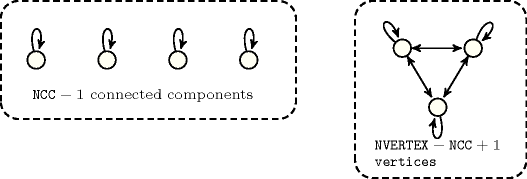Proof 98 (133) We proceed by induction on $T\left(G\right)=\mathrm{𝐍𝐕𝐄𝐑𝐓𝐄𝐗}\left(G\right)-|X|-\left(\mathrm{𝐍𝐂𝐂}\left(G\right)-1\right)$, where $X$ is any connected component of $G$ of maximum cardinality. For $T\left(G\right)=0$ then either $\mathrm{𝐍𝐂𝐂}\left(G\right)=1$ and thus the formula is clearly true, or all the connected components of $G$, but possibly $X$, are reduced to one element. Since isolated vertices are not allowed, the formula holds.

Assume that $T\left(G\right)\ge 1$. Then there exists $Y$, a connected component of $G$ distinct from $X$, with more than one vertex. Let $y\in Y$ and let ${G}^{\text{'}}$ be the graph such that $V\left({G}^{\text{'}}\right)=V\left(G\right)$ and $E\left({G}^{\text{'}}\right)$ is defined by:

• For all $Z$ connected components of $G$ distinct from $X$ and $Y$ we have ${G}^{\text{'}}\left[Z\right]=G\left[Z\right]$.

• With ${X}^{\text{'}}=X\cup \left\{y\right\}$ and ${Y}^{\text{'}}=Y-\left\{y\right\}$, we have ${G}^{\text{'}}\left[{Y}^{\text{'}}\right]=G\left[{Y}^{\text{'}}\right]$ and $E\left({G}^{\text{'}}\left[{X}^{\text{'}}\right]\right)=E\left(G\left[X\right]\right)\cup \left({\bigcup }_{x\in {X}^{\text{'}}}\left\{\left(x,y\right),\left(y,x\right)\right\}\right)$.

Clearly $|E\left({G}^{\text{'}}\right)|-|E\left(G\right)|\ge 2·|X|+1-\left(2·|Y|-1\right)$ and since $X$ is of maximal cardinality the difference is strictly positive. Now as $\mathrm{𝐍𝐕𝐄𝐑𝐓𝐄𝐗}\left({G}^{\text{'}}\right)=\mathrm{𝐍𝐕𝐄𝐑𝐓𝐄𝐗}\left(G\right)$, $\mathrm{𝐍𝐂𝐂}\left({G}^{\text{'}}\right)=\mathrm{𝐍𝐂𝐂}\left(G\right)$ and as $T\left({G}^{\text{'}}\right)=T\left(G\right)-1$ the result holds by induction hypothesis.

Proposition 99

$\mathrm{𝐍𝐀𝐑𝐂}\ge \mathrm{𝐍𝐕𝐄𝐑𝐓𝐄𝐗}-\mathrm{𝐍𝐂𝐂}$

$\begin{array}{cc}& \mathrm{𝚎𝚚𝚞𝚒𝚟𝚊𝚕𝚎𝚗𝚌𝚎}:\mathrm{𝐍𝐂𝐂}>0⇒\\ & \mathrm{𝐍𝐀𝐑𝐂}\ge \left(\mathrm{𝐍𝐕𝐄𝐑𝐓𝐄𝐗}\mathrm{mod}\mathrm{𝐍𝐂𝐂}\right)·{\left(⌊\frac{\mathrm{𝐍𝐕𝐄𝐑𝐓𝐄𝐗}}{\mathrm{𝐍𝐂𝐂}}⌋+1\right)}^{2}+\\ & \left(\mathrm{𝐍𝐂𝐂}-\mathrm{𝐍𝐕𝐄𝐑𝐓𝐄𝐗}\mathrm{mod}\mathrm{𝐍𝐂𝐂}\right)·{⌊\frac{\mathrm{𝐍𝐕𝐄𝐑𝐓𝐄𝐗}}{\mathrm{𝐍𝐂𝐂}}⌋}^{2}\end{array}$

Proof 99 (143) By induction of the number of vertices. The formula holds for one vertex. Let $G$ a graph with $n+1$ vertices $\left(n\ge 1\right)$. First assume there exists $x$ in $G$ such that $G-x$ has the same number of connected components than $G$. Since $\mathrm{𝐍𝐀𝐑𝐂}\left(G\right)\ge \mathrm{𝐍𝐀𝐑𝐂}\left(G-x\right)+1$, and by induction hypothesis $\mathrm{𝐍𝐀𝐑𝐂}\left(G-x\right)\ge \mathrm{𝐍𝐕𝐄𝐑𝐓𝐄𝐗}\left(G-x\right)-\mathrm{𝐍𝐂𝐂}\left(G-x\right)$ the result holds. Otherwise all connected components of $G$ are reduced to one vertex and the formula holds.

Proposition 100

$\mathrm{𝐍𝐀𝐑𝐂}\le \left(\mathrm{𝐍𝐕𝐄𝐑𝐓𝐄𝐗}-\mathrm{𝐍𝐒𝐂𝐂}+1\right)·\mathrm{𝐍𝐕𝐄𝐑𝐓𝐄𝐗}+\frac{\mathrm{𝐍𝐒𝐂𝐂}·\left(\mathrm{𝐍𝐒𝐂𝐂}-1\right)}{2}$

$\mathrm{𝚎𝚚𝚞𝚒𝚟𝚊𝚕𝚎𝚗𝚌𝚎}:\mathrm{𝐍𝐀𝐑𝐂}\le \mathrm{𝐍𝐒𝐂𝐂}-1+{\left(\mathrm{𝐍𝐕𝐄𝐑𝐓𝐄𝐗}-\mathrm{𝐍𝐒𝐂𝐂}+1\right)}^{2}$

##### Figure 4.3.3. Illustration of Proposition 100(145). A graph that achieves the maximum number of arcs according to a fixed number of strongly connected components as well as to a fixed number of vertices ($\mathrm{𝙽𝚂𝙲𝙲}=5,\mathrm{𝙽𝚅𝙴𝚁𝚃𝙴𝚇}=6,\mathrm{𝙽𝙰𝚁𝙲}=\left(6-5+1\right)·6+\frac{5·\left(5-1\right)}{2}=22$)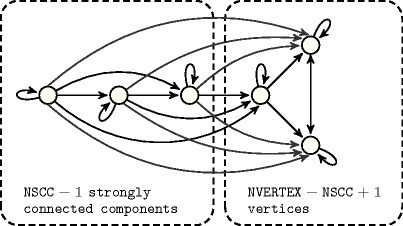Proof 100 For proving 145, it is easier to rewrite the formula as $\mathrm{𝐍𝐀𝐑𝐂}\le {\left(\mathrm{𝐍𝐕𝐄𝐑𝐓𝐄𝐗}-\left(\mathrm{𝐍𝐒𝐂𝐂}-1\right)\right)}^{2}+\left(\mathrm{𝐍𝐂𝐂}-1\right)·\left(\mathrm{𝐍𝐕𝐄𝐑𝐓𝐄𝐗}-\left(\mathrm{𝐍𝐒𝐂𝐂}-1\right)\right)+\frac{\mathrm{𝐍𝐒𝐂𝐂}·\left(\mathrm{𝐍𝐒𝐂𝐂}-1\right)}{2}$. We proceed by induction on $T\left(G\right)=\mathrm{𝐍𝐕𝐄𝐑𝐓𝐄𝐗}\left(G\right)-|X|-\left(\mathrm{𝐍𝐒𝐂𝐂}\left(G\right)-1\right)$, where $X$ is any strongly connected component of $G$ of maximum cardinality.

For $T\left(G\right)=0$ then either $\mathrm{𝐍𝐒𝐂𝐂}\left(G\right)=1$ and thus the formula is clearly true, or all the strongly connected components of $G$, but possibly $X$, are reduced to one element. Since the maximum number of arcs in a directed acyclic graph of $n$ vertices is $\frac{n·\left(n+1\right)}{2}$, and as the subgraph of $G$ induced by all the strongly connected components of $G$ excepted $X$ is acyclic, the formula clearly holds.

Assume that $T\left(G\right)\ge 1$, let ${\left({X}_{i}\right)}_{i\in I}$ be the family of strongly connected components of $G$, and let ${G}_{r}$ be the reduced graph of $G$ induced by ${\left({X}_{i}\right)}_{i\in I}$ (that is $V\left({G}_{r}\right)=I$ and $\forall {i}_{1},{i}_{2}\in I$, $\left({i}_{1},{i}_{2}\right)\in E\left({G}_{r}\right)$ if and only if $\exists {x}_{1}\in {X}_{{i}_{1}}$, $\exists {x}_{2}\in {X}_{{i}_{2}}$ such that $\left({x}_{1},{x}_{2}\right)\in E$). Consider ${G}^{\text{'}}$ such that $V\left({G}^{\text{'}}\right)=V\left(G\right)$ and $E\left({G}^{\text{'}}\right)$ is defined by:

• For all strongly connected components $Z$ of $G$ we have ${G}^{\text{'}}\left[Z\right]=G\left[Z\right]$.

• For $\sigma$ be any topological sort of ${G}_{r}$, $\forall {x}_{i}\in {X}_{i}$, $\forall {x}_{j}\in {X}_{j}$, $\left({x}_{i},{x}_{j}\right)\in E\left({G}^{\text{'}}\right)$ whenever $i$ is less than $j$ with respect to $\sigma$.

Notice that ${G}^{\text{'}}$ satisfies the following properties: $T\left({G}^{\text{'}}\right)=T\left(G\right)$, $V\left({G}^{\text{'}}\right)=V\left(G\right)$, $\mathrm{𝐍𝐒𝐂𝐂}\left({G}^{\text{'}}\right)=\mathrm{𝐍𝐒𝐂𝐂}\left(G\right)$, $E\left(G\right)\subseteq E\left({G}^{\text{'}}\right)$, ${\left({X}_{i}\right)}_{i\in I}$ is still the family of strongly connected components of ${G}^{\text{'}}$, and moreover, for every $i\in I$ and every ${x}_{i}\in {X}_{i}$ we have that ${x}_{i}$ is connected to any vertex outside ${X}_{i}$, that is the number of arcs incident to ${x}_{i}$ and incident to vertices outside ${X}_{i}$ is exactly $|V\left({G}^{\text{'}}\right)|-|{X}_{i}|$.

Now, as $T\left({G}^{\text{'}}\right)\ge 1$, there exists $Y$, a strongly connected component of ${G}^{\text{'}}$ distinct from $X$, with more than one vertex. Let $y\in Y$ and let ${G}^{\text{'}\text{'}}$ be the graph such that $V\left({G}^{\text{'}\text{'}}\right)=V\left({G}^{\text{'}}\right)$ and $E\left({G}^{\text{'}\text{'}}\right)$ is defined by:

• ${G}^{\text{'}\text{'}}\left[V\left(G\right)-\left\{y\right\}\right]={G}^{\text{'}}\left[V\left(G\right)-\left\{y\right\}\right]$.

• With ${X}^{\text{'}}=X\cup \left\{y\right\}$, we have ${G}^{\text{'}\text{'}}\left[{Y}^{\text{'}}\right]={G}^{\text{'}}\left[{Y}^{\text{'}}\right]$ and $E\left({G}^{\text{'}\text{'}}\left[{X}^{\text{'}}\right]\right)=E\left({G}^{\text{'}}\left[X\right]\right)\cup \left({\bigcup }_{x\in {X}^{\text{'}}}\left\{\left(x,y\right),\left(y,x\right)\right\}\right)$.

• Assume that $X={X}_{j}$ for $j\in I$. Then $\forall i\in I-\left\{j\right\}$, $\forall {x}_{i}\in {X}_{i}$, $\left({x}_{i},y\right)\in E\left({G}^{\text{'}\text{'}}\right)$ whenever $i$ is less than $j$ with respect to $\sigma$ and $\left(y,{x}_{i}\right)\in E\left({G}^{\text{'}\text{'}}\right)$ whenever $j$ is less than $i$ with respect to $\sigma$.

Clearly $|E\left({G}^{\text{'}\text{'}}\right)|-|E\left({G}^{\text{'}}\right)|\ge 2|X|+1+|V\left({G}^{\text{'}}\right)|-|X|-\left(2·|Y|-1+|V\left({G}^{\text{'}}\right)|-|Y|\right)=|X|-|Y|+2$ and since $X$ is of maximal cardinality the difference is strictly positive. As $E\left(G\right)\subseteq E\left({G}^{\text{'}}\right)$, $|E\left({G}^{\text{'}\text{'}}\right)|-|E\left(G\right)|$ is also strictly positive. Now as $\mathrm{𝐍𝐕𝐄𝐑𝐓𝐄𝐗}\left({G}^{\text{'}\text{'}}\right)=\mathrm{𝐍𝐕𝐄𝐑𝐓𝐄𝐗}\left({G}^{\text{'}}\right)=\mathrm{𝐍𝐕𝐄𝐑𝐓𝐄𝐗}\left(G\right)$, $\mathrm{𝐍𝐒𝐂𝐂}\left({G}^{\text{'}\text{'}}\right)=\mathrm{𝐍𝐒𝐂𝐂}\left({G}^{\text{'}}\right)=\mathrm{𝐍𝐒𝐂𝐂}\left(G\right)$ and as $T\left({G}^{\text{'}\text{'}}\right)=T\left({G}^{\text{'}}\right)-1=T\left(G\right)-1$ the result holds by induction hypothesis.

Proposition 101

$\mathrm{𝐍𝐀𝐑𝐂}\ge \mathrm{𝐍𝐕𝐄𝐑𝐓𝐄𝐗}-⌊\frac{\mathrm{𝐍𝐒𝐂𝐂}-1}{2}⌋$

$\begin{array}{cc}& \mathrm{𝚎𝚚𝚞𝚒𝚟𝚊𝚕𝚎𝚗𝚌𝚎}:\mathrm{𝐍𝐒𝐂𝐂}>0⇒\\ & \mathrm{𝐍𝐀𝐑𝐂}\ge \left(\mathrm{𝐍𝐕𝐄𝐑𝐓𝐄𝐗}\mathrm{mod}\mathrm{𝐍𝐒𝐂𝐂}\right)·{\left(⌊\frac{\mathrm{𝐍𝐕𝐄𝐑𝐓𝐄𝐗}}{\mathrm{𝐍𝐒𝐂𝐂}}⌋+1\right)}^{2}+\\ & \left(\mathrm{𝐍𝐒𝐂𝐂}-\mathrm{𝐍𝐕𝐄𝐑𝐓𝐄𝐗}\mathrm{mod}\mathrm{𝐍𝐒𝐂𝐂}\right)·{⌊\frac{\mathrm{𝐍𝐕𝐄𝐑𝐓𝐄𝐗}}{\mathrm{𝐍𝐒𝐂𝐂}}⌋}^{2}\end{array}$

##### Figure 4.3.4. Illustration of Proposition 147. A graph that achieves the minimum number of arcs according to a fixed number of strongly connected components as well as to a fixed number of vertices ($\mathrm{𝙽𝚂𝙲𝙲}=7,\mathrm{𝙽𝚅𝙴𝚁𝚃𝙴𝚇}=10,\mathrm{𝙽𝙰𝚁𝙲}=10-⌊\frac{7}{2}⌋=7$)Proof 101 For proving part 147 of Proposition 101 we proceed by induction on $\mathrm{𝐍𝐒𝐂𝐂}\left(G\right)$. If $\mathrm{𝐍𝐒𝐂𝐂}\left(G\right)=1$ then, we have $\mathrm{𝐍𝐀𝐑𝐂}\left(G\right)\ge \mathrm{𝐍𝐕𝐄𝐑𝐓𝐄𝐗}\left(G\right)$ (i.e., for one vertex this is true since every vertex has at least one arc, otherwise every vertex $v$ has an arc arriving on $v$ as well as an arc starting from $v$, thus we have $\mathrm{𝐍𝐀𝐑𝐂}\ge \frac{2·\mathrm{𝐍𝐕𝐄𝐑𝐓𝐄𝐗}}{2}$). If $\mathrm{𝐍𝐒𝐂𝐂}\left(G\right)>1$ let $X$ be a strongly connected component of $G$. Then $\mathrm{𝐍𝐀𝐑𝐂}\left(G\right)\ge \mathrm{𝐍𝐀𝐑𝐂}\left(G\left[V\left(G\right)-X\right]\right)+\mathrm{𝐍𝐀𝐑𝐂}\left(G\left[X\right]\right)$. By induction hypothesis $\mathrm{𝐍𝐀𝐑𝐂}\left(G\left[V\left(G\right)-X\right]\right)\ge |V\left(G\right)-X|-⌊\frac{\mathrm{𝐍𝐒𝐂𝐂}\left(G\left[V\left(G\right)-X\right]\right)-1}{2}⌋$, thus $\mathrm{𝐍𝐀𝐑𝐂}\left(G\left[V\left(G\right)-X\right]\right)\ge |V\left(G\right)-X|-⌊\frac{\left(\mathrm{𝐍𝐒𝐂𝐂}\left(G\right)-1\right)-1}{2}⌋$. Since $\mathrm{𝐍𝐀𝐑𝐂}\left(G\left[X\right]\right)\ge |X|$ we obtain $\mathrm{𝐍𝐀𝐑𝐂}\left(G\right)\ge |V\left(G\right)|-⌊\frac{\left(\mathrm{𝐍𝐒𝐂𝐂}\left(G\right)-1\right)-1}{2}⌋$, and thus the result holds.

Proposition 102

$\mathrm{𝚎𝚚𝚞𝚒𝚟𝚊𝚕𝚎𝚗𝚌𝚎}:\mathrm{𝐍𝐕𝐄𝐑𝐓𝐄𝐗}>0⇒\mathrm{𝐍𝐒𝐂𝐂}\ge ⌈\frac{{\mathrm{𝐍𝐕𝐄𝐑𝐓𝐄𝐗}}^{2}}{\mathrm{𝐍𝐀𝐑𝐂}}⌉$

Proof 102 As shown in [BessiereHebrardHnichKiziltanWalsh05], a lower bound for the minimum number of equivalence classes (e.g., strongly connected components) is the independence number of the graph and the right-hand side of Proposition 102 corresponds to a lower bound of the independence number proposed by Turán

Proposition 103

$\mathrm{𝚎𝚚𝚞𝚒𝚟𝚊𝚕𝚎𝚗𝚌𝚎}:\mathrm{𝐍𝐕𝐄𝐑𝐓𝐄𝐗}>0⇒\mathrm{𝐍𝐒𝐂𝐂}\ge ⌈\frac{2·\mathrm{𝐍𝐕𝐄𝐑𝐓𝐄𝐗}-\frac{\mathrm{𝐍𝐀𝐑𝐂}-\mathrm{𝐍𝐕𝐄𝐑𝐓𝐄𝐗}}{⌈\frac{\mathrm{𝐍𝐀𝐑𝐂}-\mathrm{𝐍𝐕𝐄𝐑𝐓𝐄𝐗}}{\mathrm{𝐍𝐕𝐄𝐑𝐓𝐄𝐗}}⌉}}{⌈\frac{\mathrm{𝐍𝐀𝐑𝐂}-\mathrm{𝐍𝐕𝐄𝐑𝐓𝐄𝐗}}{\mathrm{𝐍𝐕𝐄𝐑𝐓𝐄𝐗}}⌉+1}⌉$

Proof 103 See

Proposition 104

$\mathrm{𝐍𝐀𝐑𝐂}\le \left(\mathrm{𝐍𝐕𝐄𝐑𝐓𝐄𝐗}-\mathrm{𝐍𝐒𝐈𝐍𝐊}\right)·\mathrm{𝐍𝐕𝐄𝐑𝐓𝐄𝐗}$

Proof 104 The maximum number of arcs is achieved by the following pattern: for all non-sink vertices we have an arc to all vertices.

Proposition 105

$\mathrm{𝐍𝐀𝐑𝐂}\ge \mathrm{𝐍𝐒𝐈𝐍𝐊}+max\left(0,\mathrm{𝐍𝐕𝐄𝐑𝐓𝐄𝐗}-2·\mathrm{𝐍𝐒𝐈𝐍𝐊}\right)$

##### Figure 4.3.5. Illustration of Proposition 105. Two graphs that achieve the minimum number of arcs according to a fixed number of sinks as well as to a fixed number of vertices: (A) $\mathrm{𝙽𝚂𝙸𝙽𝙺}=3,\mathrm{𝙽𝚅𝙴𝚁𝚃𝙴𝚇}=5,\mathrm{𝙽𝙰𝚁𝙲}=3+max\left(0,5-2·3\right)=3$; (B) $\mathrm{𝙽𝚂𝙸𝙽𝙺}=3,\mathrm{𝙽𝚅𝙴𝚁𝚃𝙴𝚇}=9,\mathrm{𝙽𝙰𝚁𝙲}=3+max\left(0,9-2·3\right)=6$.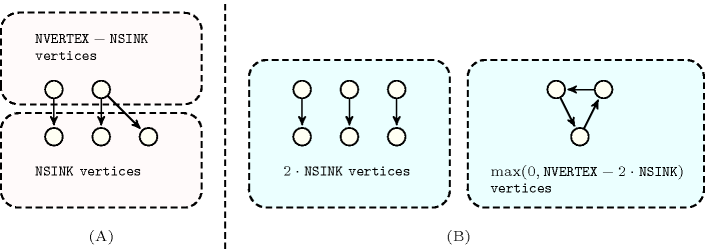Proof 105 Recall that for $x\in V\left(G\right)$, we have that ${d}_{G}^{+}\left(x\right)+{d}_{G}^{-}\left(x\right)\ge 1$. If $x$ is a sink then ${d}_{G}^{-}\left(x\right)\ge 1$, consequently $\mathrm{𝐍𝐀𝐑𝐂}\left(G\right)\ge \mathrm{𝐍𝐒𝐈𝐍𝐊}\left(G\right)$. If $x$ is not a sink then ${d}_{G}^{+}\left(x\right)\ge 1$, consequently $\mathrm{𝐍𝐀𝐑𝐂}\left(G\right)\ge |V\left(G\right)|-\mathrm{𝐍𝐒𝐈𝐍𝐊}\left(G\right)$.

Proposition 106

$\mathrm{𝐍𝐀𝐑𝐂}\le \left(\mathrm{𝐍𝐕𝐄𝐑𝐓𝐄𝐗}-\mathrm{𝐍𝐒𝐎𝐔𝐑𝐂𝐄}\right)·\mathrm{𝐍𝐕𝐄𝐑𝐓𝐄𝐗}$

Proof 106 The maximum number of arcs is achieved by the following pattern: for all non-source vertices we have an arc from all vertices.

Proposition 107

$\mathrm{𝐍𝐀𝐑𝐂}\ge \mathrm{𝐍𝐒𝐎𝐔𝐑𝐂𝐄}+max\left(0,\mathrm{𝐍𝐕𝐄𝐑𝐓𝐄𝐗}-2·\mathrm{𝐍𝐒𝐎𝐔𝐑𝐂𝐄}\right)$

##### Figure 4.3.6. Illustration of Proposition 107. Two graphs that achieve the minimum number of arcs according to a fixed number of sources as well as to a fixed number of vertices: (A) $\mathrm{𝙽𝚂𝙾𝚄𝚁𝙲𝙴}=3,\mathrm{𝙽𝚅𝙴𝚁𝚃𝙴𝚇}=5,\mathrm{𝙽𝙰𝚁𝙲}=3+max\left(0,5-2·3\right)=3$; (B) $\mathrm{𝙽𝚂𝙾𝚄𝚁𝙲𝙴}=3,\mathrm{𝙽𝚅𝙴𝚁𝚃𝙴𝚇}=9,\mathrm{𝙽𝙰𝚁𝙲}=3+max\left(0,9-2·3\right)=6$.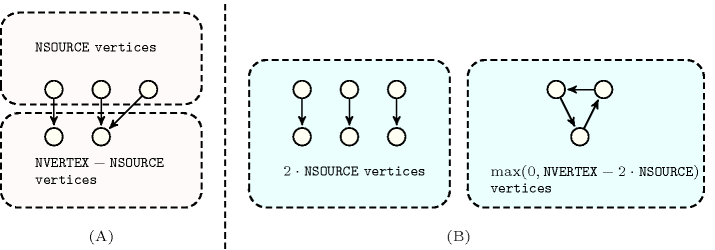Proof 107 Similar to Proposition 105.

Proposition 108

$\mathrm{𝐍𝐒𝐂𝐂}\ge \mathrm{𝐍𝐒𝐈𝐍𝐊}+\mathrm{𝐍𝐒𝐎𝐔𝐑𝐂𝐄}$

Proof 108 Since sinks and sources cannot belong to a circuit and since they cannot coincide (i.e., because isolated vertices are not allowed) the result follows.

Proposition 109

$\mathrm{𝐍𝐕𝐄𝐑𝐓𝐄𝐗}\ge \mathrm{𝐍𝐒𝐈𝐍𝐊}+\mathrm{𝐍𝐒𝐎𝐔𝐑𝐂𝐄}$

Proof 109 No vertex can be both a source and a sink (isolated vertices are removed).